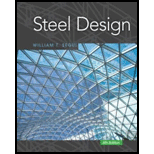# A tensile test was performed on a metal specimen with a circular cross section. The diameter was measured to be 0.550 inch. Two marks were made along the length of the specimen and were measured to be 2.030 inches apart. This distance is defined as the gage length , and all length measurements are made between the two marks. The specimen was loaded to failure. Fracture occurred at a load of 28,500 pounds. The specimen was then reassembled, and the diameter and gage length were measured to be 0.430 inch and 2.300 inches. Determine the a. Ultimate tensile stress in ksi. b. Elongation as a percentage. c. Reduction in cross-sectional area as a percentage.### Steel Design (Activate Learning wi...

6th Edition
Segui + 1 other
Publisher: Cengage Learning
ISBN: 9781337094740### Steel Design (Activate Learning wi...

6th Edition
Segui + 1 other
Publisher: Cengage Learning
ISBN: 9781337094740

#### Solutions

Chapter
Section
Chapter 1, Problem 1.5.1P
Textbook Problem

## A tensile test was performed on a metal specimen with a circular cross section. The diameter was measured to be 0.550 inch. Two marks were made along the length of the specimen and were measured to be 2.030 inches apart. This distance is defined as the gage length, and all length measurements are made between the two marks. The specimen was loaded to failure. Fracture occurred at a load of 28,500 pounds. The specimen was then reassembled, and the diameter and gage length were measured to be 0.430 inch and 2.300 inches. Determine thea. Ultimate tensile stress in ksi.b. Elongation as a percentage.c. Reduction in cross-sectional area as a percentage.

Expert Solution
To determine

(a)

The ultimate tensile stress of metal specimen.

120ksi.

### Explanation of Solution

Given:

The diameter of metal specimen is 0.550inch.

The load at facture is 28,500pounds.

Concept Used:

Write the equation to calculate the ultimate tensile stress.

f=PA ...... (I)

Here, ultimate tensile stress is f, fractured load is P, and the cross-sectional area is A.

Calculation:

Calculate the cross-sectional area of specimen.

A=π4×d2 ...... (II)

Here, diameter of the specimen is d.

Substitute 0.550inch for d in the Equation (II).

A=π4×(0.550inch)2=0.95033inch24=0.2375inch2

Calculate the ultimate tensile stress.

Substitute 28,500pounds for P and 0.2375inch2 for A in the Equation (I).

f=28,500pounds0.2375inch2×(1klb1000pounds)=28.5klb0.2375inch2=120klb/inch2×(1ksi1klb/inch2)=120ksi

Conclusion:

Thus, the ultimate tensile stress on the metal specimen is 120ksi.

Expert Solution
To determine

(b)

The elongation of the metal specimen.

13.3%.

### Explanation of Solution

Given:

The original gage length is 2.03inches.

The change in gage length is 2.3inches.

Concept Used:

Write the equation to calculate the elongation.

e=LfL0L0×100 ...... (III)

Here, the elongation is e, the length of the specimen at fracture is Lf, and the original length is L0.

Calculation:

Calculate the elongation of the metal specimen.

Substitute 2.03inches for L0 and 2.3inches for Lf in Equation (III).

e=[(2.3inches)(2.03inches)(2.03inches)]×100=0.1330×100=13.3%

Conclusion:

Thus, the elongation of the metal specimen is 13.3%.

Expert Solution
To determine

(c)

The reduction in the cross-sectional area of the metal specimen.

38.86%.

### Explanation of Solution

Given:

The original diameter of metal specimen is 0.550inch.

The diameter after fracture load is 0.430inch.

Concept Used:

Write the equation to calculate reduction in cross-sectional area.

R=A0AfA0×100 ...... (IV)

Here, the reduction in cross-sectional area is R, original cross-sectional area is A0, and the cross-sectional area after fracture load is Af.

Calculation:

Calculate the cross-sectional area after fracture load.

Substitute 0.430inch for d in the Equation (II).

A=π4×(0.430inch)2=0.5808inch24=0.1452inch2

Calculate the reduction in the cross-sectional area.

Substitute 0.1452inch2 for Af and 0.2375inch2 for A0 in Equation (IV).

R=0.2375inch20.1452inch20.2375inch2×100=0.0923inch20.2375inch2×100=0.3886×100=38.86%

Conclusion:

Thus, the reduction in the cross-sectional area is 38.86%.

### Want to see more full solutions like this?

Subscribe now to access step-by-step solutions to millions of textbook problems written by subject matter experts!

Get Solutions

### Want to see more full solutions like this?

Subscribe now to access step-by-step solutions to millions of textbook problems written by subject matter experts!

Get Solutions

## Chapter 1 Solutions

Steel Design (Activate Learning with these NEW titles from Engineering!)

Find more solutions based on key concepts
Show solutions
Use VBA to create a custom-made dialog box for Problem 14.14. 14.14 A person by the name of Huehscher developed...

Engineering Fundamentals: An Introduction to Engineering (MindTap Course List)

What capabilities should a wireless security toolkit include?

Principles of Information Security (MindTap Course List)

Neglecting the mass of the beam, compute the reactions at A and B.

International Edition---engineering Mechanics: Statics, 4th Edition

Define polymorphism, and provide three examples.

Systems Analysis and Design (Shelly Cashman Series) (MindTap Course List)

What mode is used to manually enter programs into the machine control?

Precision Machining Technology (MindTap Course List)

The access time of a hard disk can be more than 100 times faster than an SSD.

Enhanced Discovering Computers 2017 (Shelly Cashman Series) (MindTap Course List)

What is the purpose of grooving a joint before welding on thick plate?

Welding: Principles and Applications (MindTap Course List)

If your motherboard supports ECC DDR3 memory, can you substitute non-ECC DDR3 memory?

A+ Guide to Hardware (Standalone Book) (MindTap Course List)